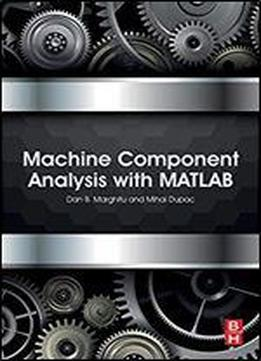# Machine Component Analysis With Matlab by Dan B. Marghitu / 2019 / English / PDF

Machine Design Analysis with MATLAB is a highly practical guide to the fundamental principles of machine design which covers the static and dynamic behavior of engineering structures and components. MATLAB has transformed the way calculations are made for engineering problems by computationally generating analytical calculations, as well as providing numerical calculations. Using step-by-step, real world example problems, this book demonstrates how you can use symbolic and numerical MATLAB as a tool to solve problems in machine design. This book provides a thorough, rigorous presentation of machine design, augmented with proven learning techniques which can be used by students and practicing engineers alike. Comprehensive coverage of the fundamental principles in machine design Uses symbolical and numerical MATLAB calculations to enhance understanding and reinforce learning Includes well-designed real-world problems and solutions

views: 602